Evolution of Young Planets

Introduction

There are two outstanding issues that one seeks to investigate by studying the evolution of a planetary core embedded in a circumstellar disk. The first regards the process of planet's migration that determines the final orbital distance from the star and, ultimately, the planet's survival. The second is the process of planetary accretion that governs the growth and therefore the final mass of the planet. The combination of the timescales over which these two phenomena occur is likely to constrain the formation timescale of the planet itself.
The discussion that follows is based on results obtained with fully three-dimensional high-resolution hydrodynamical simulations of circumstellar disks hosting planets of different masses.

Gravitational Torques and Planetary Migration

The gravitational action of a forming planet forces the circumstellar disk material to assume a characteristic configuration. This depends on how and where the perturbing waves launched by the planet are absorbed by the surrounding matter. The typical density patterns that arise from this interaction process can be seen in the two images below. These were obtained by performing a 3D simulation of a disk containing a 2 Jupiter mass (MJ) planet. The figure on the left shows the surface density of the disk whereas the one on the right displays a vertical cut of the density distribution through the planet. [Click on the images to enlarge].

 Surface Density Vertical Density Cut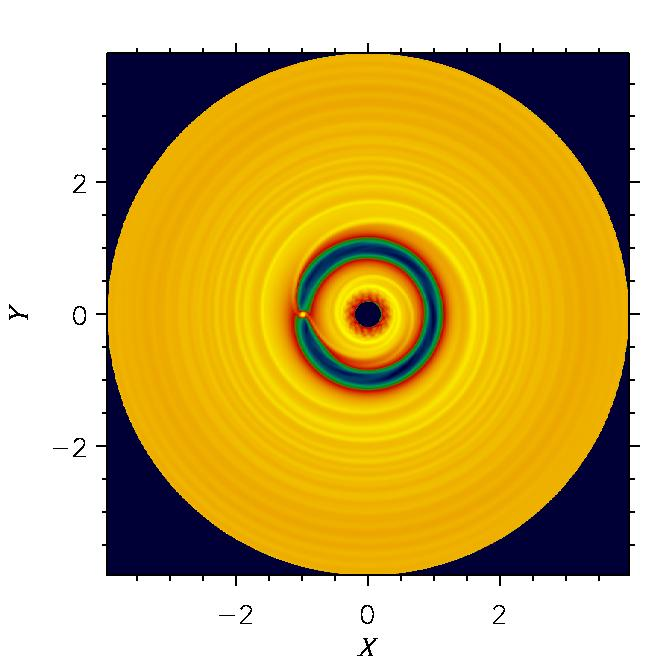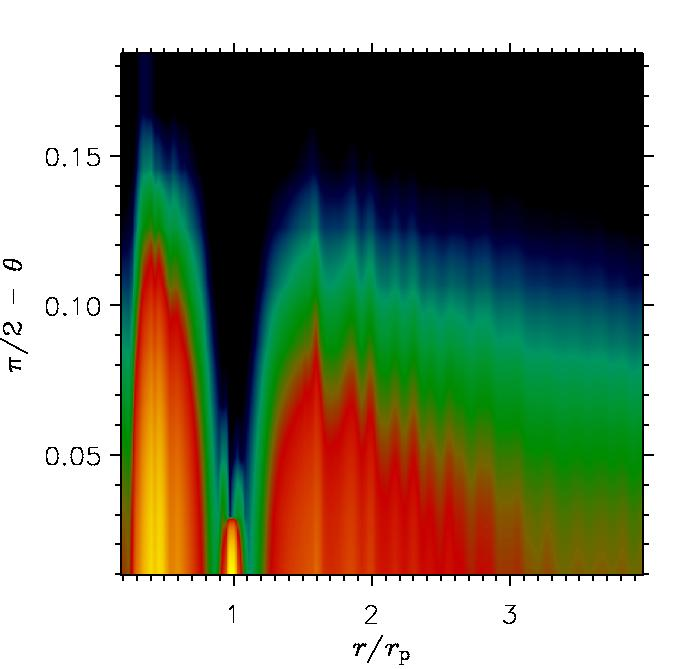Yet, gravitational perturbations induced by the planet trigger the response of the disk material that exerts an equal force onto the planet. Since perturbations are non-axisymmetric, there is a net force acting on the planet which translates into a net gravitational torque, as illustrated below. [Click on the figures to enlarge].

 Radial Torque Distribution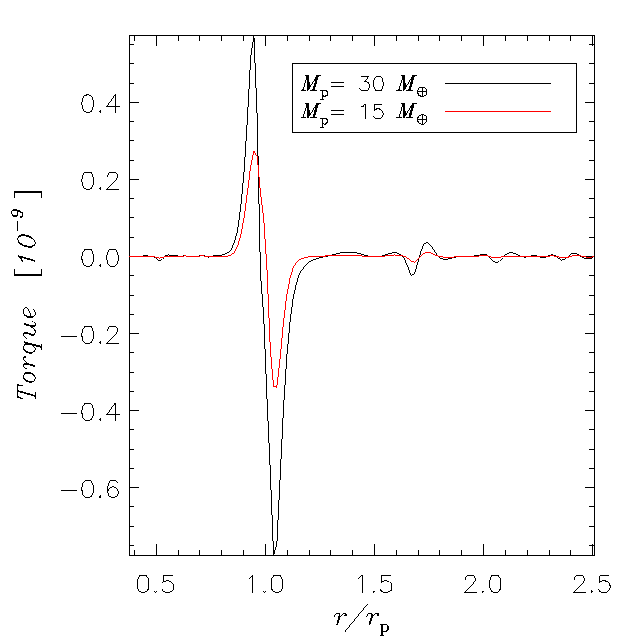These plots show the torque exerted on the planet by each disk ring as function of its radial position. The larger the disturbance caused by the planet (i.e., the larger its mass), the stronger the response of the disk is, as can be seen in the panels above.
If a net torque is exerted on the planet then its orbital angular momentum (i.e., the semi-major axis of the orbit) either increases or decreases, depending on the sign of the net torque. The variation of the orbital distance due to the exchange of angular momentum is called planet's migration. As also hinted by the torque distributions displayed above, the net torque is negative and this turns out to be often the case.

 Migration Rates The magnitude of the net torque is related to the mass of the perturber, as indicated by the figure below. The inverse of the migration timescale is plotted for a wide range of planet's masses. This quantity is proportional to the magnitude of the net torque acting on the planet.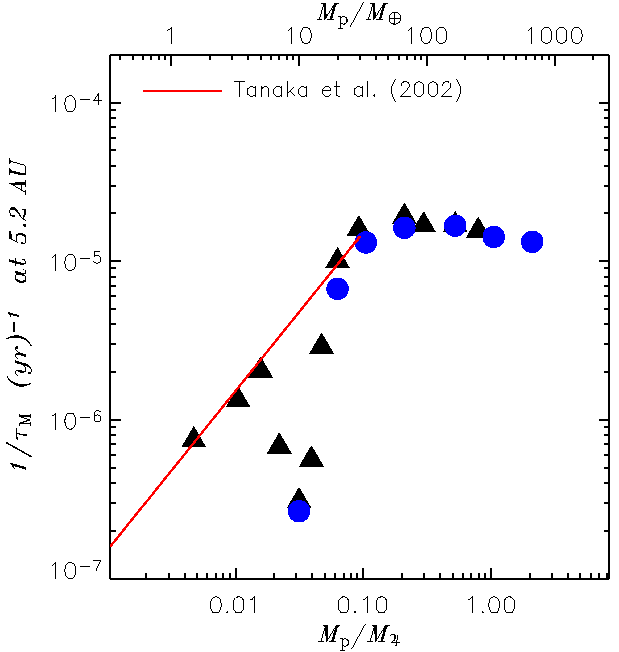The flat part rightward of 50 Earth masses, is usually referred to as Type II migration regime and it corresponds to the presence of a well developed gap along the orbit of the planet. Toward lower masses, according to analytical theories the migration should scale linearly with the planetary mass (Type I migration). Numerical data shown in the figure agree only partially with such prediction due to the onset of non-linear effects. [Click on the figure to enlarge].

Planetary Accretion

The rate at which a planet grows is determined by the amount of matter available in the surrounding region and by the strength of its gravitational field. On one hand, Jupiter-sized objects generate a deep potential well along with a wide gap, therefore the mean density is fairly low and so is the accretion rate. On the other hand, Earth mass objects have a weak gravitational field hence, although the background density can be rather high, they also accretion at very low rates. As indicated by the figures below, the maximum of the accretion rate is reached around a Saturn mass (0.3 MJ), when the gravitational field is strong enough yet the gap is not too deep and wide. [Click on the figures to enlarge].

 Accretion Rates Growth Timescale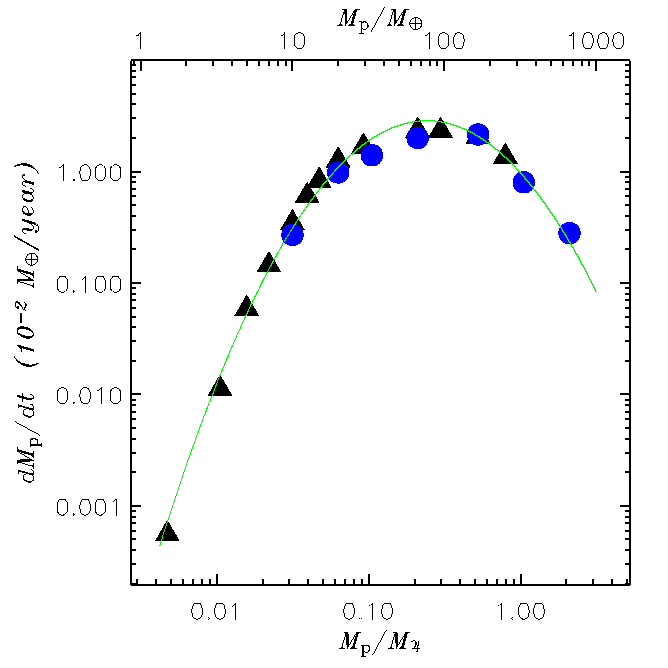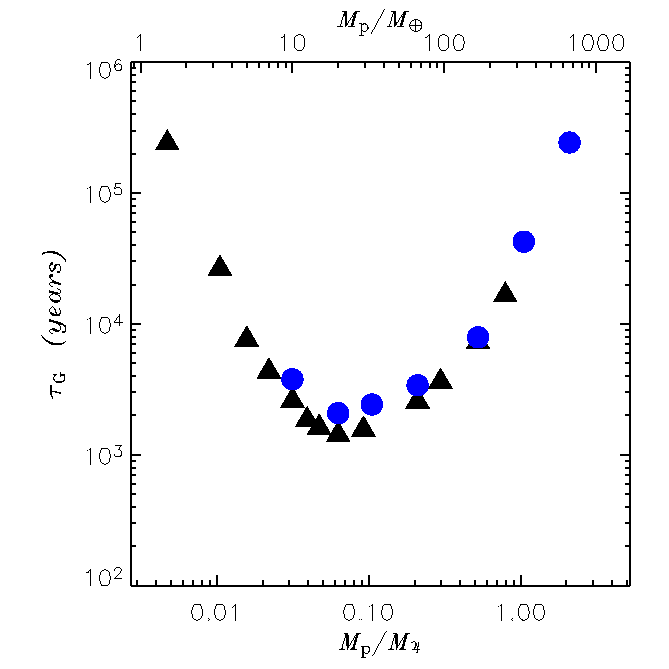The symbols with different color in the figures above refer to models where the structure of the planet was accounted for in different manners. The green line in the left panel represents a fit to the data. The growth timescale indicates the time that it takes for the mass of the planet to become 2.7 times as large.